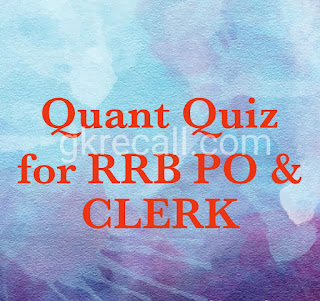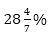# gkrecall

## QUANT SET 19 FOR RRB PO & CLERK PRE-2020RRB Clerk and RRB PO Prelims exam are going to be held in the upcoming months. We have already provided you with the PDFs of many topics of Quantitative Aptitude like Simplification/Approximation, Number Series,datainterpretation.important Arithmetic questions to prepare for RRB Clerk and PO Pre exam. Practicing these questions will help you to know about the level of the questions. To increase your speed and accuracy, enhance your calculations.

### START QUIZ

Q1. A and B entered into partnership investing Rs.24000 and Rs.32000 respectively. After 8 months, C also joins the business with capital of Rs. 30000. The share of C in a profit of Rs. 45600 after 2 years will be:
(a) 24000
(b) 32000
(c) 18000
(d) 15650
(e) None of these

Q2. When the price of vegetable oil was increased by 20%, a family reduced its consumption in such a way that the expenditure on oil is only increased by 15%. If 24 litres were consumed per month before the increase in price, find the new monthly consumption.
(a) 22 litres
(b) 23 litres
(c) 23.5 litres
(d) 22. 5 litres
(e) None of these

Q3. A person covered 18 km at 3km/h. 50 km at 5 km/h and 60 km at 10 km/h. Then find the average speed in covering the whole distance.
(a) 10 (1/11) km/ h
(b) 5 (9/11) km/ h
(c) 7.5 km/ h
(d) 6 km/h
(e) None of these

Q4. A dishonest dealer sells the goods at 10% loss on cost price but uses 20% less weight. What is his percentage profit a loss?
(a) 12.5% gain
(b) 12.5% loss
(e) None of these

Q5. Two pies A and B can fill a tank in 30 minutes and 45minutes respectively. Both pipes are opened. The tank will be filled in just 20 minutes, if the pipe B is turned off after:
(a) 10 minutes
(b) 18 minutes
(c) 8 minutes
(d) 15 minutes
(e) None of these

Q6. Rehan can do a work withmore efficiency than that of Gaffar. Gaffar and Raheem can do a work in 12 days together while Raheem alone can complete the same work in 18 days. In what time Rehan alone can complete the same work?
(a) 36 days
(b) 28 days
(c) 24 days
(d) 18 days
(e) 21 days
Q7. Marks obtained by Shobhit in Science ismore than that by Pihu in Sociology and marks obtained by Pihu in Sociology is 20% more than that by Akash in Hindi. If marks obtained by Akash in Hindi is 75% and maximum marks in each subject is 80, then find the average of marks obtained by Shobhit, Pihu and Akash in their respective subjects.
(a) 76
(b) 72
(c) Can’t be determined
(d) 78
(e) 74

Q8. Mr. Roy bought a plot @ 400 rupees per sq. feet. After sometimes, he sold 40% of the plot @ 450 rupees per sq. feet and rest @ 500 rupees sq. feet. If dimensions of plot are 25 feet × 30 feet, then find his total profit in this transaction.
(a) Rs. 80,000
(b) Rs. 72,000
(c) Rs. 50,000
(d) Rs. 60,000
(e) None of these

Q9. The compound interest and simple interest on a certain sum at certain rate of interest for two years are Rs. 2580 and Rs. 2400 respectively. What will be simple interest on same sum at same rate of interest for 6 years?
(a) Rs. 6200
(b) Rs. 7200
(c) Rs. 8400
(d) Rs. 6400
(e) Rs. 9600

Q10. In an 80 litre mixture of sugar and alcohol, alcohol is 40% and rest is sugar solution. If some amount of mixture is replaced by alcohol, the ratio of alcohol to sugar solution becomes 5: 3. Find the amount of mixture replaced by alcohol.
(a) 30 li.
(b) 40 li.
(c) 50 li.
(d) 15 li.
(e) 20 li.

Solutions

S1. Ans. (c)
Sol.

S2. Ans. (b)
Sol.

S3. Ans. (b)
Sol.

S4. Ans. (a)
Sol.

S5. Ans. (d)
Sol.

S6. Ans. (b)
Sol.

S7. Ans. (a)
Sol.

S8. Ans. (d)
Sol.

S9. Ans. (b)
Sol.

S10. Ans. (a)
Sol.